# How to Remove Duplicates in Excel

If our data is having large no. of data with duplicate entries, manual deletion would be a very big task and annoyance.

Excel is having in-built option to remove the duplicate entries from data. Remove Duplicate option is under the Data tab in the Data Tools group in Microsoft Excel 2010 and 2013.

Example

Let’s take an example and understand how we can use the Remove Duplicate option.

We have data in range A2:E13. In which column A contains month, column B contains Agent name, column C contains Country name, column D contains sales and column E contains Quantity.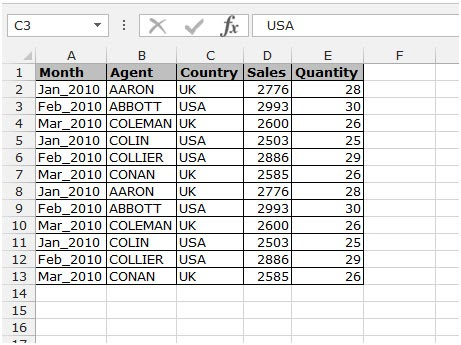Data is having duplicate entries. To remove the duplicate entries from data follow below mentioned steps:-

• Go to Data Tab, click on Remove Duplicates in Data Tools group.
•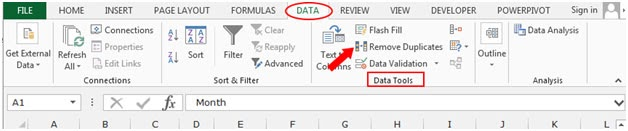• Remove Duplicates dialog box will appear.
•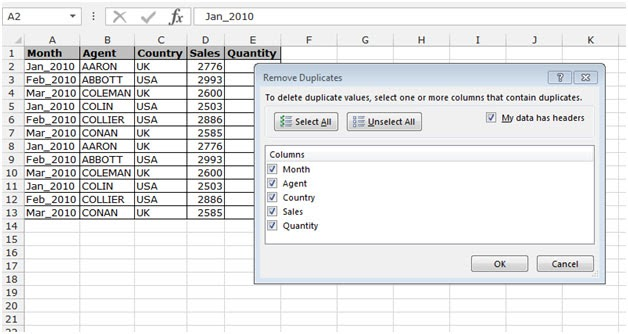• In the column box select the columns header on which basis you want to remove duplicate entries.
• In this data we want to remove duplicate entries on the agent name, we just select the Agent.
•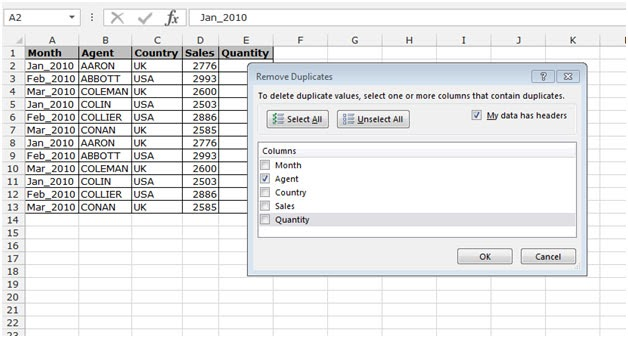• After select the criteria as per the requirement of data click on ok.
• You will get an information popup to inform you that how many duplicates entries were found and how many unique values are left now.
•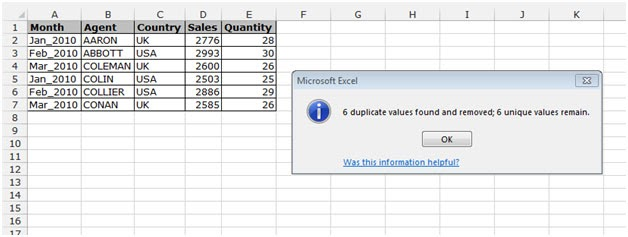This is the way you can remove duplicate entries from the large no. of data and can get the data with unique records.

Alternate idea

When a list contains a lot of values and we needed to confirm the list contains duplicate values or not. For this we will make a combination of excel functions and use the formula to get the desired result. Use the SUMPRODUCT function & COUNTIF function.

SUMPRODUCT function is a mathematical function in Excel. It operates on multiple ranges. It multiplies the corresponding arrays and then adds them.

Syntax:

 = SUMPRODUCT ( list1, [list2], … )

COUNTIF function of excel just counts the number of cells with a specific condition in a given range.

Syntax:

 = COUNTIF ( list, condition )

Formation of the formula:

First we need to check every element of the list with every other element of the same list. Then we will set a limit on the count by subtracting 1 from each occurrence and returns the required result.

 = SUMPRODUCT ( COUNTIF ( list, list ) -1 ) > 0

Example:

Let’s understand this formula using in the example shown below.

For this example, We took some names as a list in excel as shown below.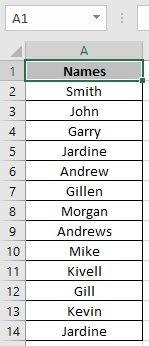And we need to find if there's a duplicate value or not.

Use the Formula

 = SUMPRODUCT ( COUNTIF( A2:A14 , A2:A14 ) -1 ) > 0

Explanation :

COUNTIF function counts the occurrence of each value in the list and returns an array of numbers, where number represents their counts

{ 1 ; 1 ; 1 ; 2 ; 1 ; 1 ; 1 ; 1 ; 1 ; 1 ; 1 ; 1 ; 2 }

Subtract 1 from each value in the list.

{ 0 ; 0 ; 0 ; 1 ; 0 ; 0 ; 0 ; 0 ; 0 ; 0 ; 0 ; 0 ; 1 }

Now the SUMPRODUCT function adds up all the value and returns TRUE if the result is greater than 1 or else it returns FALSE.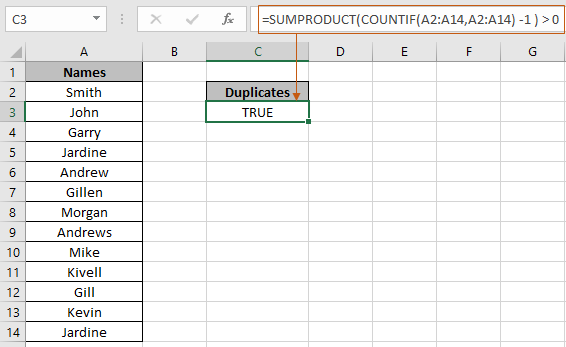In the above snapshot the formula used to get the duplicates in a list in excel.

Blank cells in the list, may cause the formula to generate incorrect results. To ignore blank cells in the list use the below formula.

Use the formula if the list contains blank cells

 = SUMPRODUCT ( ( COUNTIF ( list , list ) - 1 ) * ( list < > " " ) ) > 0

Here are some observational notes shown below.

Notes:

1. The formula only works with numbers and text both.
2. You can apply the formula over any column of the table where condition can be applied.
3. Careful working with fixed reference and absolute array reference.
4. Operators like equals to ( = ), less than equal to ( <= ), greater than ( > ) or not equals to ( <> ) can be performed within functions applied with numbers only.

Hope this article about How to Remove Duplicates in Excel is explanatory. Find more articles on Count formulas and related Excel tools here. If you liked our blogs, share it with your friends on Facebook. And also you can follow us on Twitter and Facebook. We would love to hear from you, do let us know how we can improve, complement or innovate our work and make it better for you. Write to us at info@exceltip.com.

Related Articles

How to use the SUMPRODUCT function in Excel: Returns the SUM after multiplication of values in multiple arrays in excel. This function can be used to do multiple tasks. This is one of the most versatile functions.

COUNTIFS with Dynamic Criteria Range : To count with dynamic criteria range we simply use the INDIRECT function. This function can

COUNTIFS With OR For Multiple Criteria : Count cells having multiple criteria match using the OR function. To put an OR logic in COUNTIFS function you will not need to use the OR function.

Using the IF with AND / OR Functions in Microsoft Excel : These logical functions are used to carry out multiple criteria calculations. With IF the OR and AND functions are used to include or exclude matches.

How to use OR function in Microsoft Excel : The function is used to include all the TRUE values in multiple criterias.

How to Count Cells That Contain This Or That in Excel in Excel :To cells that contain this or that, we can use the SUMPRODUCT function. Here's how you do those calculations.

Popular Articles :

How to use the IF Function in Excel : The IF statement in Excel checks the condition and returns a specific value if the condition is TRUE or returns another specific value if FALSE.

How to use the VLOOKUP Function in Excel : This is one of the most used and popular functions of excel that is used to lookup value from different ranges and sheets.

How to Use SUMIF Function in Excel : This is another dashboard essential function. This helps you sum up values on specific conditions.

How to use the COUNTIF Function in Excel : Count values with conditions using this amazing function. You don't need to filter your data to count specific values. Countif function is essential to prepare your dashboard.

Terms and Conditions of use

The applications/code on this site are distributed as is and without warranties or liability. In no event shall the owner of the copyrights, or the authors of the applications/code be liable for any loss of profit, any problems or any damage resulting from the use or evaluation of the applications/code.